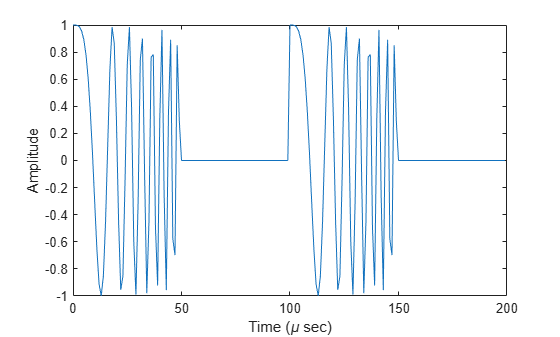# step

System object: phased.LinearFMWaveform
Package: phased

Samples of linear FM pulse waveform

## Syntax

Y = step(sLFM) Y = step(sLFM,prfidx) Y = step(sRFM,freqoffset) [Y,PRF] = step(___) [Y,COEFF] = step(___) 

## Description

Note

Starting in R2016b, instead of using the step method to perform the operation defined by the System object™, you can call the object with arguments, as if it were a function. For example, y = step(obj,x) and y = obj(x) perform equivalent operations. When the only argument to the step method is the System object itself, replace y = step(obj) by y = obj().

Y = step(sLFM) returns samples of the linear FM pulse in a column vector Y.

Y = step(sLFM,prfidx), uses the prfidx index to select the PRF from the predefined vector of values specified by in the PRF property. This syntax applies when you set the PRFSelectionInputPort property to true.

Y = step(sRFM,freqoffset), uses the freqoffset to generate the waveform with an offset as specified at step time. Use this syntax for cases where the transmit pulse frequency needs to be dynamically updated. This syntax applies when you set the FrequencyOffsetSource property to 'Input port'.

[Y,PRF] = step(___) also returns the current pulse repetition frequency, PRF. To enable this syntax, set the PRFOutputPort property to true and set the OutputFormat property to 'Pulses'.

[Y,COEFF] = step(___) returns the matched filter coefficients, COEFF, for the current pulse. To enable this syntax, set the CoefficientsOutputPort property to true. COEFF is returned as either an NZ-by-1 vector or an NZ-by-M matrix.

• An NZ-by-1 vector is returned when:

• The object has OutputFormat set to 'Pulses' and NumPulses is equal to 1. NZ is the pulse width.

• The object is configured to generate constant pulse width waveforms (DurationSpecification is set to 'Pulse width' or 'Duty cycle' and PRF has one unique value); and either OutputFormat is set to 'Pulses' and NumPulses is greater than 1, or the OutputFormat is set to 'Samples'. For this case, NZ is the pulse width.

• An NZ-by-M matrix is returned when the object generates varying pulse widths (DurationSpecification property is set to 'Duty cycle' and PRF has more than one unique value); and either OutputFormat set to 'Pulses' and NumPulses is greater than 1, or OutputFormat is set to 'Samples'. For this case, NZ is the maximum of the pulse widths, and M is the number of unique PRFs.

You can combine optional input and output arguments when their enabling properties are set. Optional inputs and outputs must be listed in the same order as the order of the enabling properties. For example, [Y,PRF,COEFF] = step(sRFM,prfidx,freqoffset).

Note

The object performs an initialization the first time the object is executed. This initialization locks nontunable properties and input specifications, such as dimensions, complexity, and data type of the input data. If you change a nontunable property or an input specification, the System object issues an error. To change nontunable properties or inputs, you must first call the release method to unlock the object.

## Examples

expand all

Construct a linear FM waveform having a sweep bandwidth of 300 kHz, a sample rate of 1 MHz, a pulse width of 50 microseconds, and a pulse repetition frequency of 10 kHz. Generate two pulses.

sLFM = phased.LinearFMWaveform('SweepBandwidth',3e5,... 'OutputFormat','Pulses','SampleRate',1e6,... 'PulseWidth',50e-6,'PRF',10e3,'NumPulses',2);

Obtain and plot the linear FM waveform.

wav = step(sLFM); numpulses = size(wav,1); t = [0:(numpulses-1)]/sLFM.SampleRate; plot(t*1e6,real(wav)) xlabel('Time (\mu sec)') ylabel('Amplitude')Construct six linear FM waveform pulses having a sweep bandwidth of 300 kHz, a sample rate of 1 MHz, a pulse width of 50 microseconds, and a duty cycle of 20%. Vary the pulse repetition frequency.

Set the sample rate and PRF. The ratio of sample rate to PRF must be an integer.

fs = 1e6; PRF = [10000,25000]; sLFM = phased.LinearFMWaveform('SweepBandwidth',3e5,... 'OutputFormat','Pulses','SampleRate',fs,... 'DurationSpecification','Duty Cycle','DutyCycle',.2,... 'PRF',PRF,'NumPulses',1,'PRFSelectionInputPort',true);

Obtain and plot the linear FM waveforms. For the first three calls to the step method, set the PRF to 10 kHz using the PRF index. For the next three calls, set the PRF to 25 kHz.

wav = []; for n = 1:6 idx = floor((n-1)/3)+1; wav1 = step(sLFM,idx); wav = [wav;wav1]; end nsamps = size(wav,1); t = [0:(nsamps-1)]/sLFM.SampleRate; plot(t*1e6,real(wav)) xlabel('Time (\mu sec)') ylabel('Amplitude')Generate output samples and matched filter coefficients of a linear FM pulse waveform at a 50 kHz frequency offset.

waveform = phased.LinearFMWaveform('SweepBandwidth',1e5, ... 'PulseWidth',5e-5,'OutputFormat','Pulses', ... 'FrequencyOffset',5e4,'CoefficientsOutputPort',true); [wav,coeff] = waveform();

Create a matched filter that applies the coefficients as an input argument. Use the coefficients when applying the matched filter to the waveform. Plot the waveform and matched filter outputs.

mf = phased.MatchedFilter('CoefficientsSource','Input port'); mfOut = mf(wav,coeff); subplot(211),plot(real(wav)); xlabel('Samples'),ylabel('Amplitude'),title('Waveform Output'); subplot(212),plot(abs(mfOut)); xlabel('Samples'),ylabel('Amplitude'),title('Matched Filter Output');